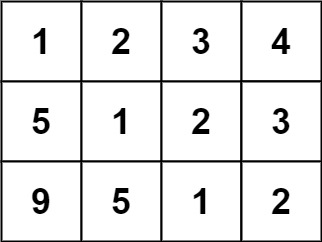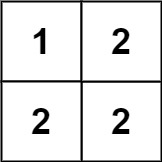# GeetCode Hub

Given an `m x n` `matrix`, return `true` if the matrix is Toeplitz. Otherwise, return `false`.

A matrix is Toeplitz if every diagonal from top-left to bottom-right has the same elements.

Example 1:```Input: matrix = [[1,2,3,4],[5,1,2,3],[9,5,1,2]]
Output: true
Explanation:
In the above grid, the diagonals are:
"", "[5, 5]", "[1, 1, 1]", "[2, 2, 2]", "[3, 3]", "".
In each diagonal all elements are the same, so the answer is True.
```

Example 2:```Input: matrix = [[1,2],[2,2]]
Output: false
Explanation:
The diagonal "[1, 2]" has different elements.
```

Constraints:

• `m == matrix.length`
• `n == matrix[i].length`
• `1 <= m, n <= 20`
• `0 <= matrix[i][j] <= 99`

• What if the `matrix` is stored on disk, and the memory is limited such that you can only load at most one row of the matrix into the memory at once?
• What if the `matrix` is so large that you can only load up a partial row into the memory at once?